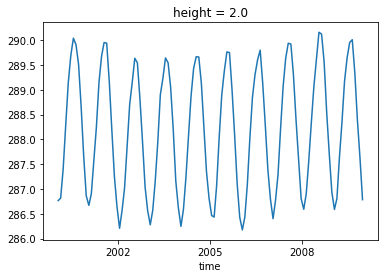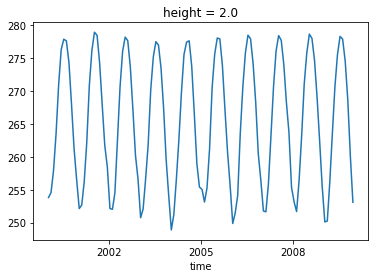Example Weighted/masked average

# Example: Masked and weighted average:

This example focuses on area weights (weighting by the area of the grid cell), but is generalizable.

import xarray as xr
import numpy as np
import matplotlib.pyplot as plt
# or
# from imports import (plt, np, xr)
path = '~/shared-cmip6-for-ns1000k/historical/NorESM2-LM/r1i1p1f1/tas_Amon_NorESM2-LM_historical_r1i1p1f1_gn_200001-200912.nc'
tas_ds = xr.open_dataset(path)


## Get the area weigts:

For the CMIP data, each model has a file for the area of each grid cell. The variable name of this is 'areacella'. We load the NorESM one.

path_area_weight ='~/shared-cmip6-for-ns1000k/historical/NorESM2-LM/r1i1p1f1/areacella_fx_NorESM2-LM_historical_r1i1p1f1_gn.nc'
areacella= xr.open_dataset(path_area_weight)


## A useful functions to do a weighted and masked average

def masked_average(xa:xr.DataArray,
dim=None,
weights:xr.DataArray=None,
mask:xr.DataArray=None):
"""
This function will average
:param xa: dataArray
:param dim: dimension or list of dimensions. e.g. 'lat' or ['lat','lon','time']
:param weights: weights (as xarray)
:param mask: mask (as xarray), True where values to be masked.
:return: masked average xarray
"""
#lest make a copy of the xa
xa_copy:xr.DataArray = xa.copy()

if mask is not None:
xa_weighted_average = __weighted_average_with_mask(
dim, mask, weights, xa, xa_copy
)
elif weights is not None:
xa_weighted_average = __weighted_average(
dim, weights, xa, xa_copy
)
else:
xa_weighted_average =  xa.mean(dim)

return xa_weighted_average

# %% [markdown]

def __weighted_average(dim, weights, xa, xa_copy):
'''helper function for masked_average'''
_, weights_all_dims = xr.broadcast(xa, weights)  # broadcast to all dims
x_times_w = xa_copy * weights_all_dims
xw_sum = x_times_w.sum(dim)
x_tot = weights_all_dims.where(xa_copy.notnull()).sum(dim=dim)
xa_weighted_average = xw_sum / x_tot
return xa_weighted_average

def __weighted_average_with_mask(dim, mask, weights, xa, xa_copy):
'''helper function for masked_average'''
_, mask_all_dims = xr.broadcast(xa, mask)  # broadcast to all dims
xa_copy = xa_copy.where(np.logical_not(mask))
if weights is not None:
_, weights_all_dims = xr.broadcast(xa, weights)  # broadcast to all dims
weights_all_dims = weights_all_dims.where(~mask_all_dims)
x_times_w = xa_copy * weights_all_dims
xw_sum = x_times_w.sum(dim=dim)
x_tot = weights_all_dims.where(xa_copy.notnull()).sum(dim=dim)
xa_weighted_average = xw_sum / x_tot
else:
xa_weighted_average = xa_copy.mean(dim)
return xa_weighted_average

# ## Application 1: Weigted global average:
# Grid cells have different area, so when we do the global average, they have to be weigted by the area of each grid cell.
# Here we do it for 2 m temperature:

aw_xr = areacella['areacella']
glob_mean = masked_average(tas_ds['tas'], dim=['lat','lon'], weights=aw_xr)
glob_mean.plot()

[<matplotlib.lines.Line2D at 0x7f6e0df10d30>]## Application 1: Weigted arctic average:

Let's try to also take only the data above 60$^\circ$N

aw_xr = areacella['areacella']
# mask values with lat < 60 deg north
mask = tas_ds['lat']<60.
glob_mean = masked_average(tas_ds['tas'], dim=['lat','lon'], weights=aw_xr, mask=mask)
glob_mean.plot()

[<matplotlib.lines.Line2D at 0x7f6e09c53470>]